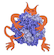IMP  2.4.0 The Integrative Modeling Platform
IMP::kernel::DerivativeAccumulator Class Reference

Class for adding derivatives from restraints to the model. More...

#include <IMP/kernel/DerivativeAccumulator.h>

Detailed Description

Class for adding derivatives from restraints to the model.

This class was created so that restraints can be weighted using a RestraintSet and that the derivatives would be scaled appropriately

Definition at line 25 of file kernel/DerivativeAccumulator.h.

Public Member Functions

DerivativeAccumulator (double weight=1.0)
the weight is one by default More...

DerivativeAccumulator (const DerivativeAccumulator &copy, double weight)
The weight is multiplied by the new weight. More...

double get_weight () const

double operator() (const double value) const
Scale a value appropriately. More...

void show (std::ostream &out=std::cout) const

Constructor & Destructor Documentation

 IMP::kernel::DerivativeAccumulator::DerivativeAccumulator ( double weight = 1.0 )

the weight is one by default

Definition at line 29 of file kernel/DerivativeAccumulator.h.

 IMP::kernel::DerivativeAccumulator::DerivativeAccumulator ( const DerivativeAccumulator & copy, double weight )

The weight is multiplied by the new weight.

Definition at line 32 of file kernel/DerivativeAccumulator.h.

Member Function Documentation

 double IMP::kernel::DerivativeAccumulator::operator() ( const double value ) const

Scale a value appropriately.

Parameters
 [in] value Value to add to the float attribute derivative.

Definition at line 38 of file kernel/DerivativeAccumulator.h.Here is the call graph for this function:

The documentation for this class was generated from the following file: